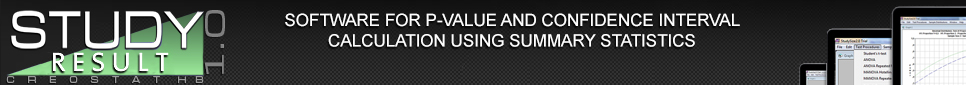### Tests and Other Procedures

 Distributions and parameters included in version 1.0. In this case the sample is assumed to come from a certain statistical distribution with an unknown parameter. The intention is to perform a test or calculate a confidence interval for the parameter. The p-value and the width of the confidence interval is then calculated using the appropriate summary statistics. The following distributions and parameters are available. Normal distribution Means (standard deviation known) Means (standard deviation unknown) Standard deviation (mean known) Standard deviation (mean unknown) Lognormal distribution Median (shape known) Median (shape unknown) Exponential distribution Location (scale known) Scale (Location known) Scale (Location unknown) Binomial distribution Proportion Odds Hypergeometric distribution Proportion Poisson distribution Mean Hazard rate Finite population Population mean Population proportion Bivariate normal distribution Mean 2 -Mean 1 Standard deviation 2 / Standard deviation 1 Correlation coefficient Regression coefficient Ā